# huangx607087学习分组密码的笔记 1

## 1. DES

### 1o01 什么是DES

DES是一种使用$56$位密钥对$64$位长分组进行加密的密码，这也是一种对称密码，也就是其加密和解密使用相同的密钥，因此DES也是一种迭代算法。DES对铭文中每个分组的加密过程都包含16轮，且每一轮的操作完全相同，每一轮的密钥都不同，但每一轮的密钥都是由上一轮的密钥中推导出来的。

DES加密中，主要思想还是混淆与扩散：混淆是将密钥与密文之间的关系尽可能模糊——因此多数情况下的混淆就是元素替换。而扩散是为了隐藏明文的统计属性，从而将一个明文符号扩散道多个密文符号进行加密，其中最简单的一个方法就是某几位上进行置换。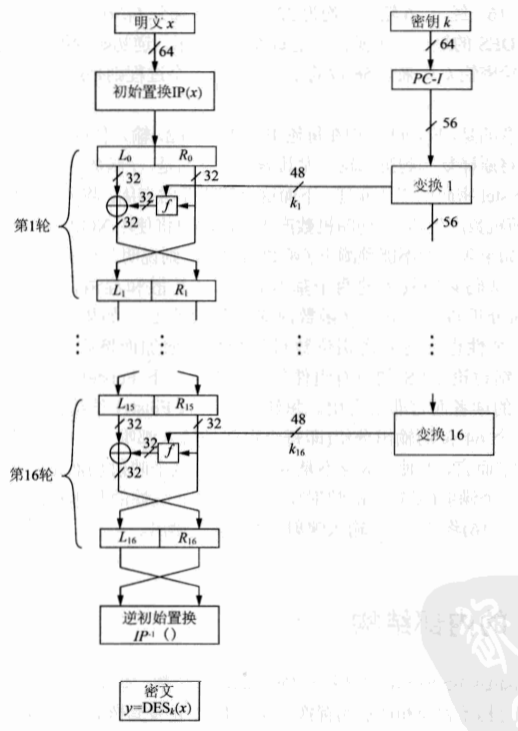### 1o02 DES内部加密分析

#### Step 1 IP置换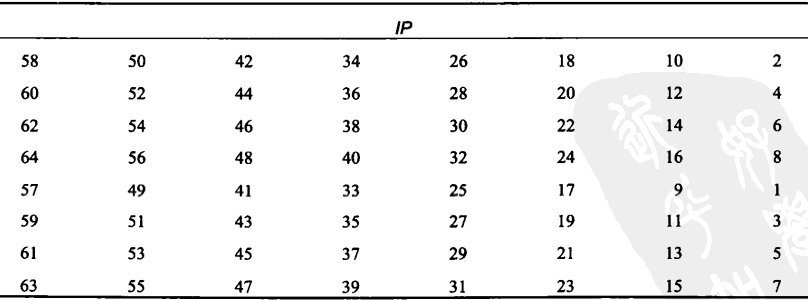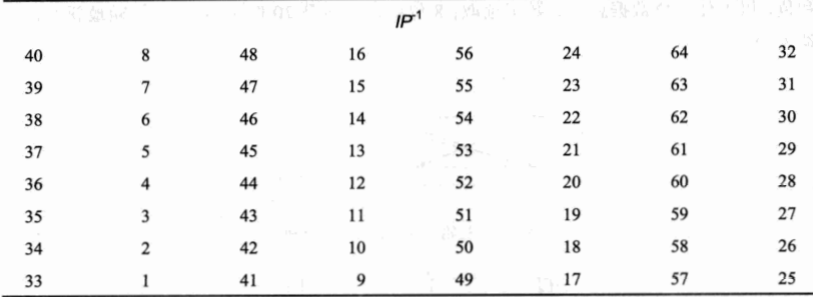#### Step 2 $f$函数

$f$函数内部结构比较复杂，如图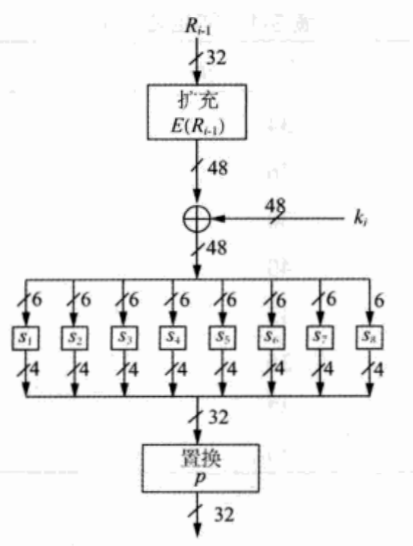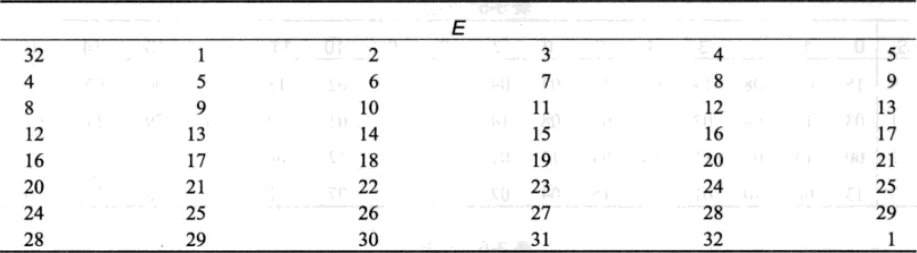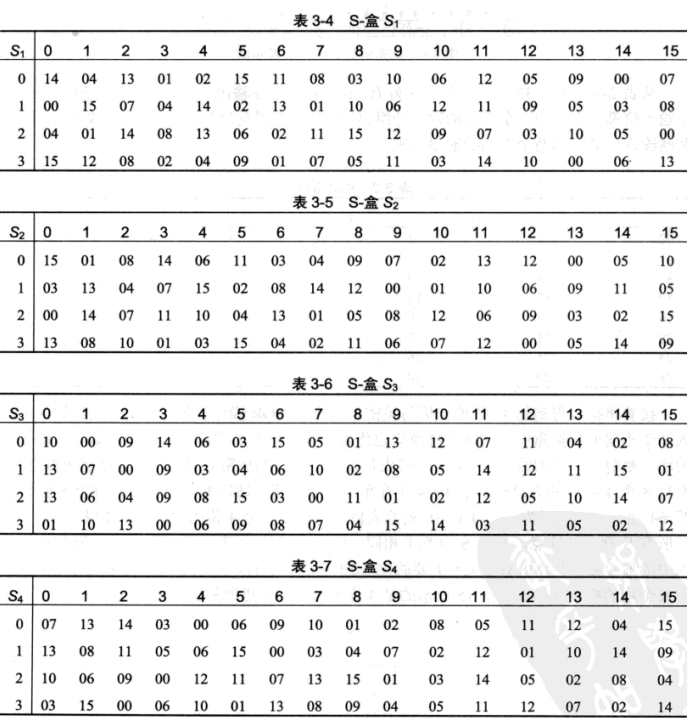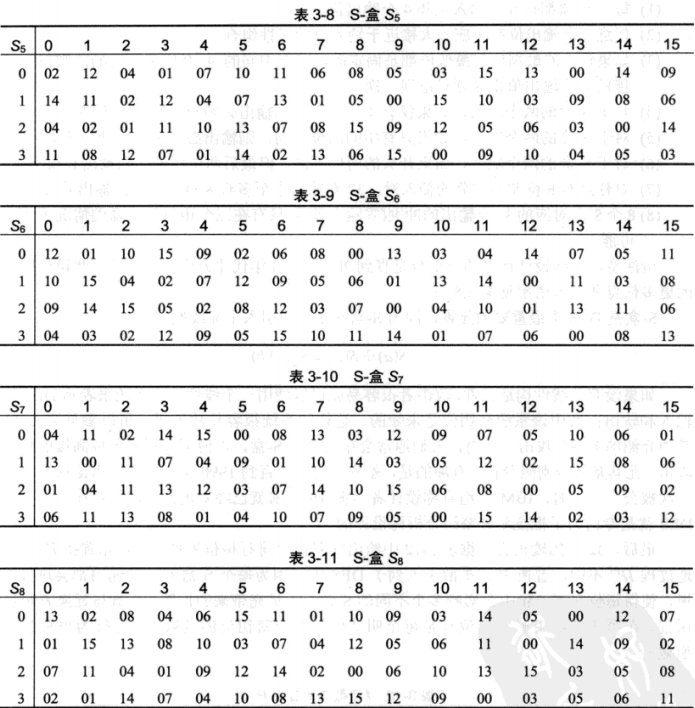$s$盒也是DES中唯一的非线性元素，因为$s(a)+s(b)$与$s(a+b)$不同。（此处的加号表示异或）

### 1o03 DES密钥编排

DES的密钥编排，是先从原始的$56$位密钥中得到$16$个轮密钥$k_1,k_2,…k_{16}$，每个密钥$k_i$都是$48$位。不过在这里一个值得注意的特点就是：DES一开始的输入密钥是$64$位，八位一组，每一组的第八位是这一组的一个校验位（但这一位的意义何在不得而知）。因此实际上严格地说，DES实际上是$56$位，而不是$64$位。因为在密钥置换的时候，$64$位缩短为$56$位时，直接忽略了每一组的第八位。下表是DES初始密钥置换的表格。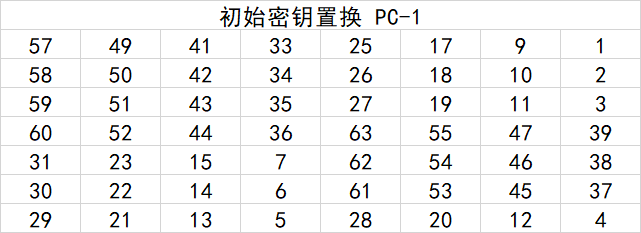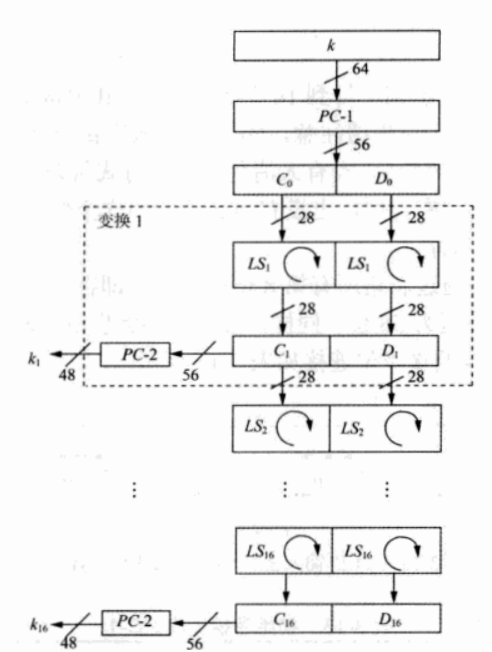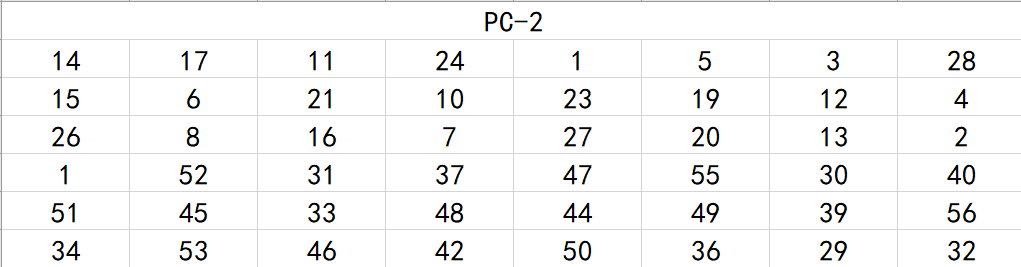### 1o04 DES解密过程$$k_{15}=\mathrm{PC_2}(C_ {15},D_ {15})=\mathrm{PC_2}(\mathrm{ror}(C_ {0}),\mathrm{ror}(D_ {0}))=\mathrm{PC_2}(\mathrm{ror}(C_ {16}),\mathrm{ror}(D_ {16}))$$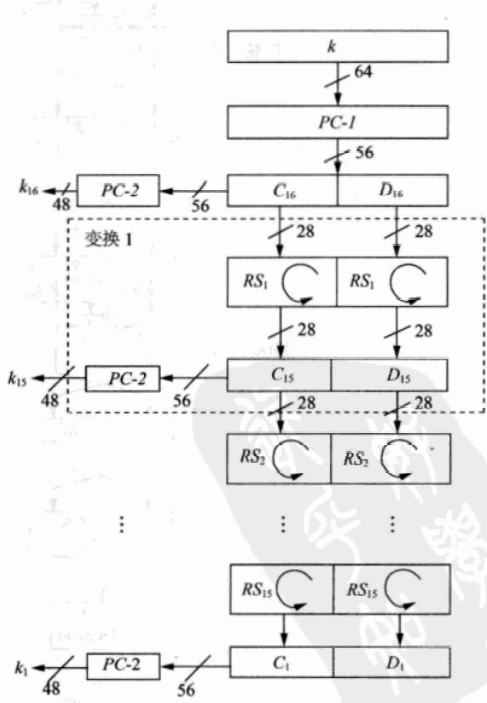$$(L_{D0},R_{D0})=\mathrm{IP}(\mathrm{IP}^{-1}(R_{16},L_{16}))=(R_{16},L_{16})$$

$$(L_{D0},R_{D0})=(R_{16},L_{16})=(R_{16},R_{15})$$

$$L_{D1}=R_{D0}=L_{16}=R_{15}$$

$$R_{D1}=L_{D0}+f(R_{D0},k_{16})=R_{16}+f(L_{16},k_{16})$$

$$R_{D1}=L_{15}+f(R_{15},k_{16})+f(L_{16},k_{16})$$

$$R_{D1}=L_{15}$$

$$(L_{Di},R_{Di})=(R_{16-i},L_{16-i})$$

目录